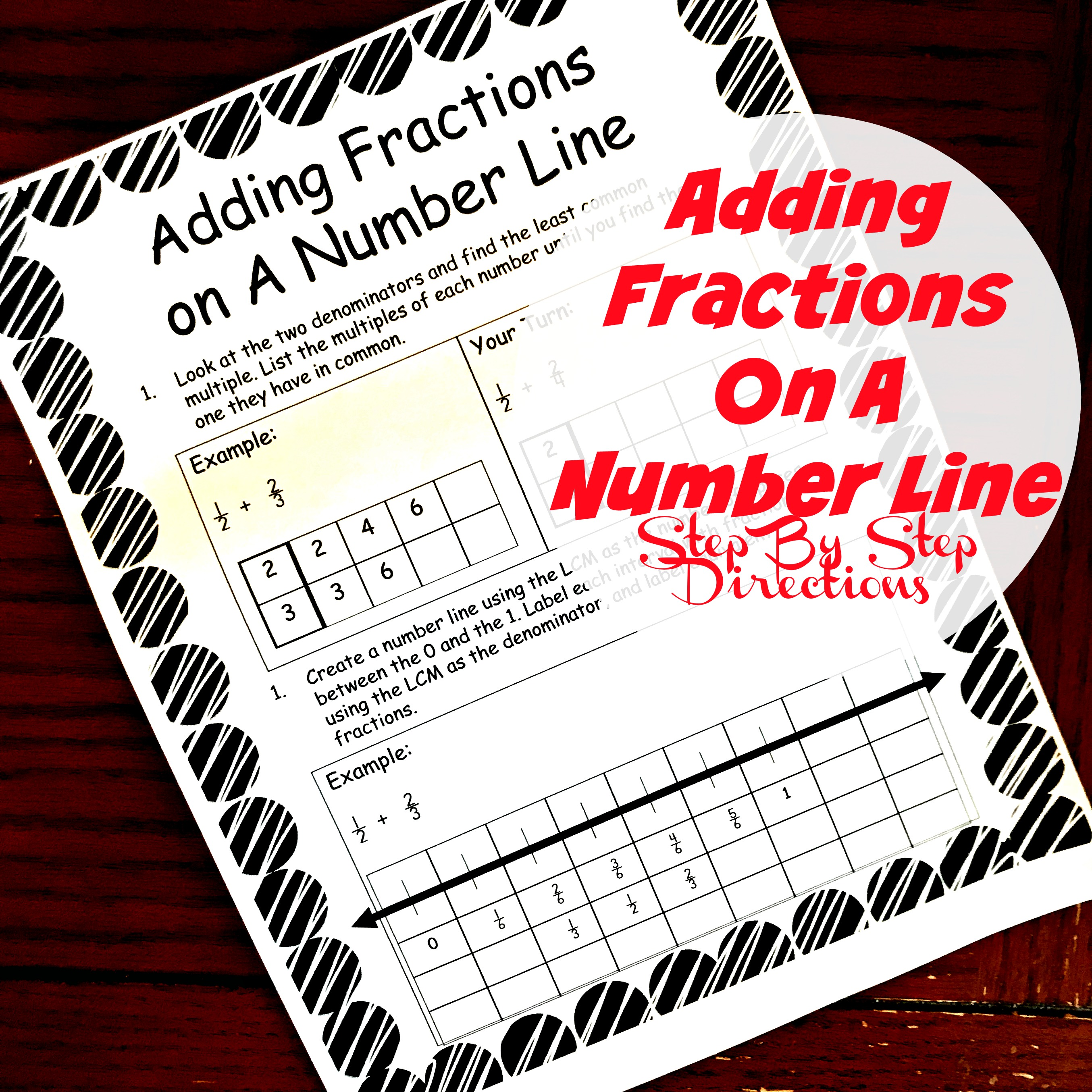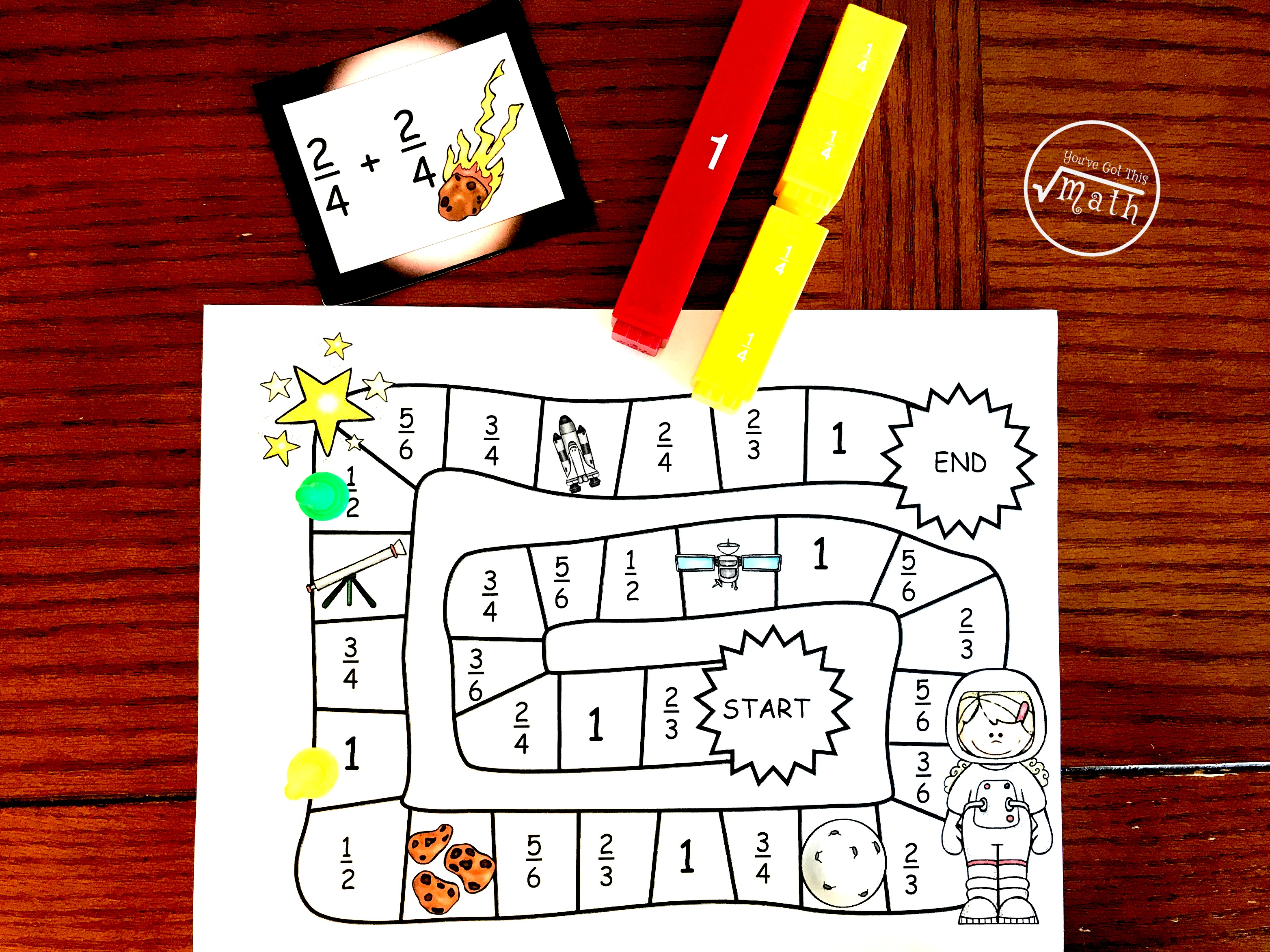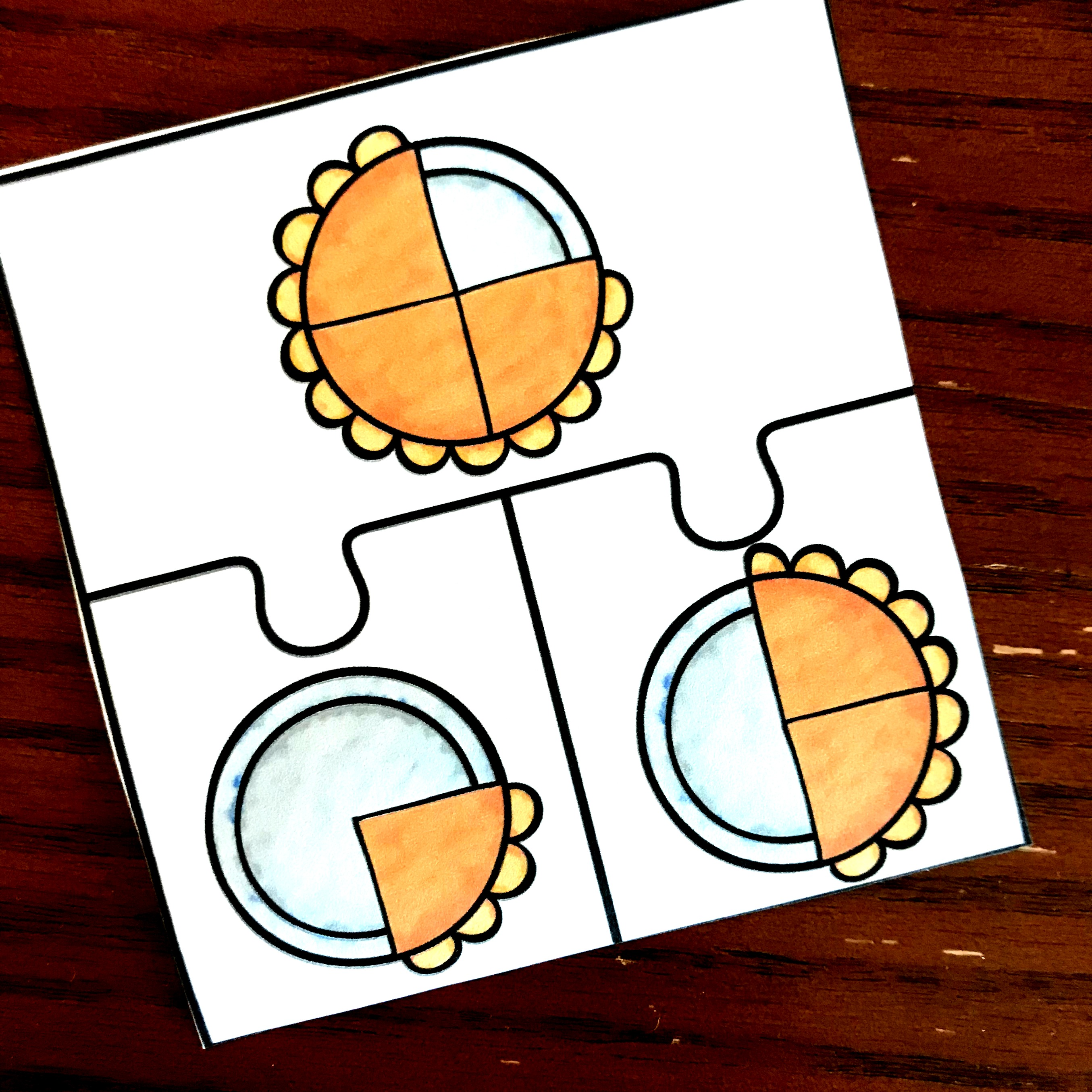Home » Math » FREE Worksheets for Adding Fractions With Unlike Denominators on a Number Line

FREE Worksheets for Adding Fractions With Unlike Denominators on a Number Line

These three FREE worksheets will help your students with adding fractions with unlike denominators on a number line. Spaces to draw line included.Adding fractions can be a challenge, but we can help our kiddos understand what they are doing and be successful by offering them visual help.

Before we talk about how to add fractions on a number line, let’s clear up one quick question many people ask. Why teach them this way.? It is so time consuming. If I just teach them to change the denominators and add, it will be much quicker.

Yes, that is true. And our goal is to get there. But have you ever had a student add 1/2 + 1/3 and say it was 2/6. Then you know how important it is to model adding fraction using models and number lines.

After the concept is understood then you can move on to teaching the formula and let your kiddos solve that way.

You can get my whole bundle to practice adding and subtractingAdding Fraction with Common Denominators:

If children have a good understanding of fractions, this will come quite easy for them. The biggest concept to get across is that we NEVER add the denominators together. Though this seems odd to some children, once they play with fractions and model them, it can begin to make sense.In this example, the students are asked to add 1/3 and 1/3. We begin by looking at our denominators and creating a number line using that. (For more info on how to do that, check out this post.)

Once we have labeled our the number line, we can add. Starting at zero we move up 1/3 which is one space, and then another 1/3 which is one more jump. We’ve landed on 2/3 and that is our answer.

Adding Fractions with Unlike Denominators:

Life gets a little more complicated here. Because we have to demonstrate Least Common Multiples on the number line.

If you want your children to see step by step instructions on how to do this, this interactive notebook will helpStep 1:

If we are adding 1/2 + 1/3 we begin by looking at the two denominators and finding the least common multiple. We do this by listing the multiples of each number until you find the one they have in common.

2: 2, 4, 6

3: 3, 6

Six is our least common multiple!

Step 2:

Now we are going to create a number line using the LCM as the number of spaces between the 0 and the 1. Since our LCM was 6, there will be six spaces between my 0 and 1. After we create the number line, we go in and label each interval with fractions using the LCM as the denominator. Finally, we label equivalent fractions.Step 3:

Our last step is to add. By looking at my equivalent fractions I see that 1/2 = 3/6…..so I will move up three spaces. Now I can see that 1/3 is equivalent to 2/6, and this lets me know to move up two more spaces.

I’ve landed on 5/6, and that is my answer.

As we help children draw number lines, label them with equivalent fractions, and then add we are developing a fraction sense. This understanding may even allow children to perform simple addition and subtraction of fractions in their head. And that is even faster than sitting down and solving a formula!

You’ve Got This,

Rachel

You may also like:

Adding common denominators game.Adding, unlike denominators pie puzzles.